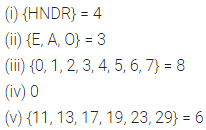# ML Aggarwal Class 6 Solutions for ICSE Maths Chapter 5 Sets Check Your Progress

## ML Aggarwal Class 6 Solutions for ICSE Maths Chapter 5 Sets Check Your Progress

Question 1.
State which of the given collections are set:
(i) Collection of all poor people of Dhanbad.
(ii) Collection of all difficult problems in your maths book.
(iii) Collection of all fools.
(iv) Collection of all countries of Asia.
(v) Collection of four countries of Asia.
(vi) Collection of three cities of India whose name start with the letter ‘J’.
(vii) Collection of all people in this world over 50 year of age.
Solution: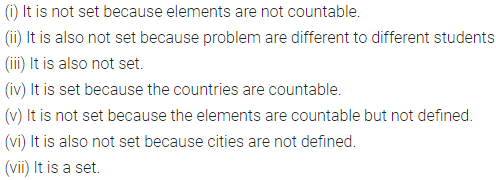Question 2.
If A = (3, 5, 7, 9, 11}, then write which of the following statements are true. If a statement is not true, mention why.
(i) 3 ϵ A
(ii) 5, 9 ϵ A
(iii) 8 ∉ A
(iv) 7 ∉ A
(v) {3} ϵ A
(vi) {5, 9} ϵ A
Solution:Question 3.
Write the following sets in the roster farm :
(i) A = (x | x is a month of a year having 30 days}
(ii) B = (x | x = 2n, n ϵ W and n < 5}
(iii) C = (x | x ϵ N and x2 < 40}
(iv) D = (all letters in the word PERMISSION}
(v) E = (x : x ϵ I and x2 < 10}
(vi) F = (x : x ϵ N, 15 < x < 50 and x is divisible by 6}
(vii) the set of whole numbers which are greater than 14 and divisible by 7.
(viii) the set of signs of four fundamental operation of arithmetic.
Solution:Question 4.
Write the following sets in set builder form :
(i) A = (2, 3, 5, 7, 11, 13, 17, 19}
(ii) B = (all months of a year}
(iii) C = (Monday, Tuesday, Wednesday}
Solution: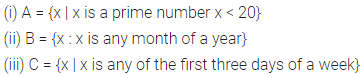Question 5.
Write the following sets in roster form and also in set builder form :
(i) A = {even whole numbers which are less than 50}
(ii) B = {two digit numbers which are perfect square}
(iii) The set of letters in the word MUSSOORIE
Solution: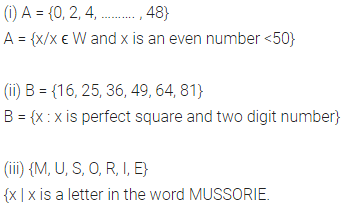Question 6.
The sets on the left are in tabular form while the sets on the right are in set builder form. Match them.
(i) {2, 3} – (a) {x/x ϵ N and x < 6}
(ii) {P, A, Y} – (b) {x: x is a prime factor of 6}
(iii) {1, 3, 5} – (c) {x | x is an odd natural number less than 6}
(iv) {1, 2, 3, 4, 5} – (d) {x/x is a letter in word PAPAYA}
Solution: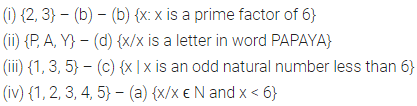Question 7.
Classify the following sets as empty set, finite set or infinite set:
(i) The set of all even prime number > 2.
(ii) The set of even prime numbers.
(iii) The set of prime numbers less than one crore.
(iv) {All points on a line segment of length 3cm}.
Solution: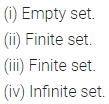Question 8.
Find the cardinal number of the following sets.
(i) A = {x | x is a consonant in the word HUNDRED}
(ii) B = {x/x is a vowel in the word DEHRADOON}
(iii) C = {x | x ϵ W and x2 < 50}